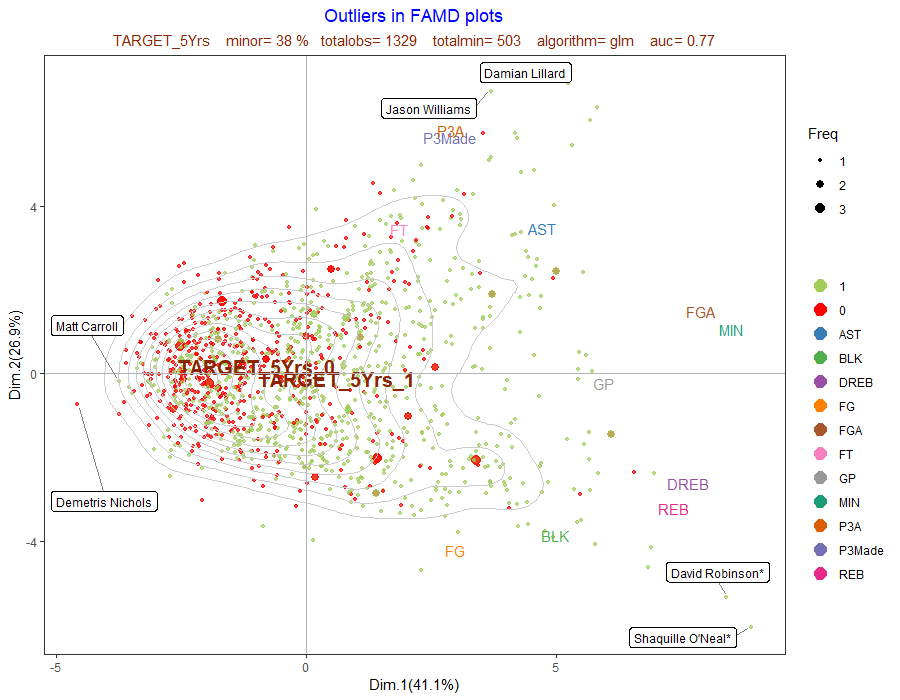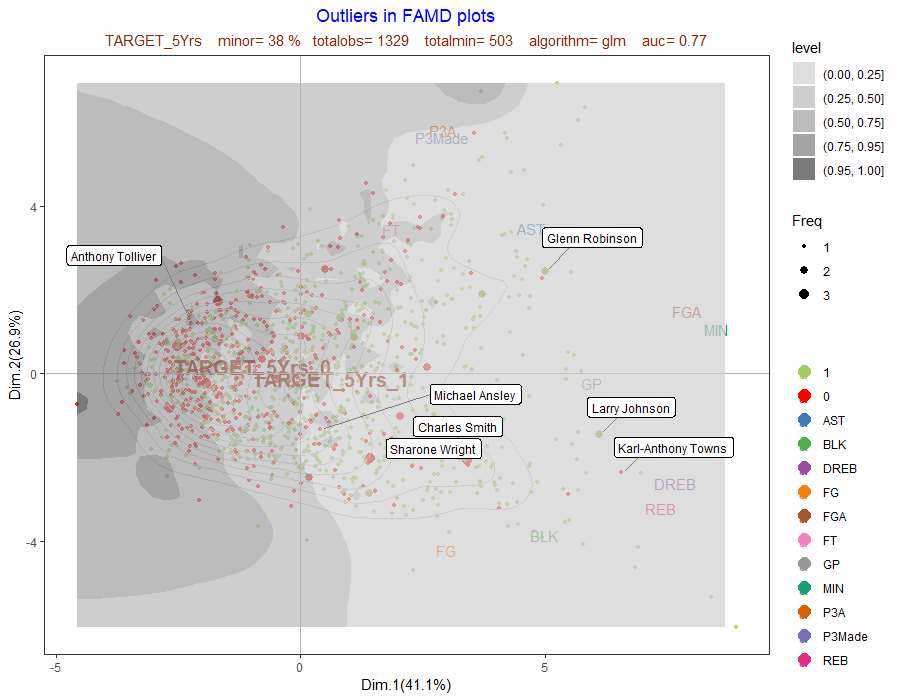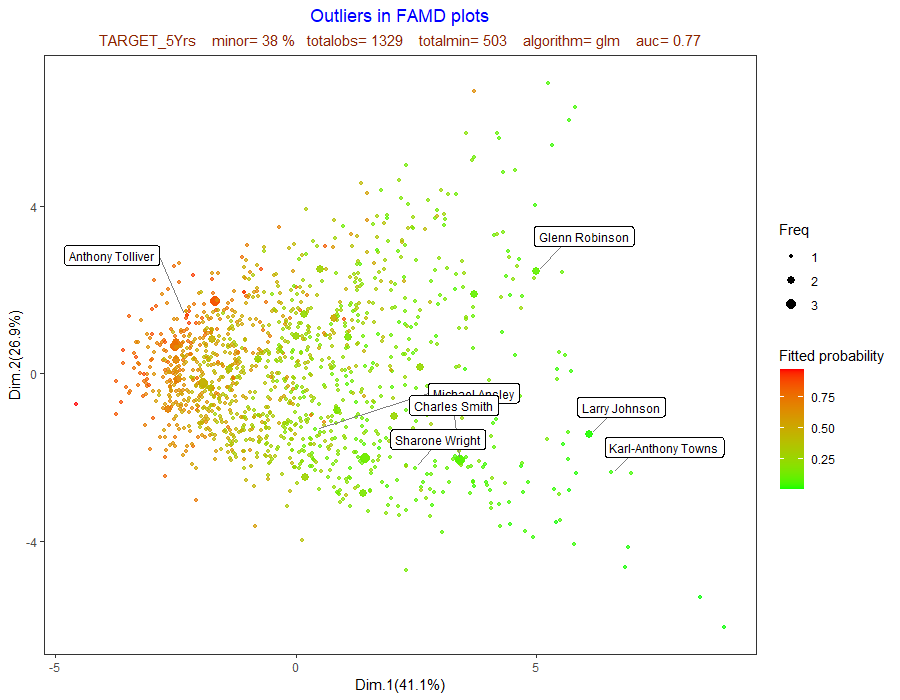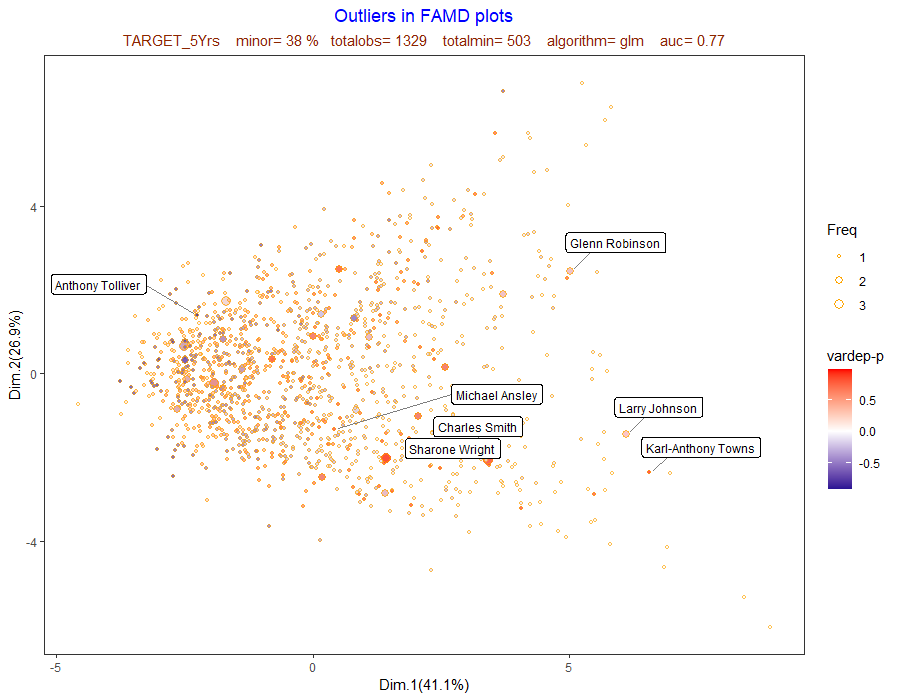# Outliers with respect to plot dimensions

In order to represent outliers, 12 plots are generated.These are the same plots as in the basic famd function, with labels for individuals that accomplish a cutpoint criteria. Idt is the label variable (Idt=“” by default for row number). For outliers with respect to the two dimensions, inf and sup cutpoints are used.

Next plot shows outliers with respect to Dim.1 and Dim.2 . It can be seen that there are players with special performance in some variables (DREB and REB for Shaquille O'Neal and David Robinson, for example).

``````library(visualpred)
dataf<-na.omit(nba)
listconti<-c("GP", "MIN", "PTS", "FGM", "FGA", "FG", "P3Made", "P3A",
"P3", "FTM", "FTA", "FT", "OREB", "DREB", "REB", "AST", "STL",
"BLK", "TOV")
listclass<-c("")
vardep<-"TARGET_5Yrs"

result<-famdcontourlabel(dataf=dataf,listconti=listconti,listclass=listclass,vardep=vardep,Idt="name",
title="Outliers in FAMD plots",title2="",selec=1,inf=0.001,sup=0.999,cutprob=0.9)
``````
``````result[]
``````# Outliers with respect to model fit

For outliers with respect to model fit, the cutpoint cutprob for the difference between vardep value (in 0,1 scale) and probability estimate is used.

Next plots show outliers with respect to model fit. Larry Johnson is fitted with high probability to green class (>5 years),but he really belongs to the red class (<5 years). Package visualpred allways codes as red the minority class; in this dataset this is TARGET_5Yrs=0.

``````result[]
````````````result[]
````````````result[]
``````